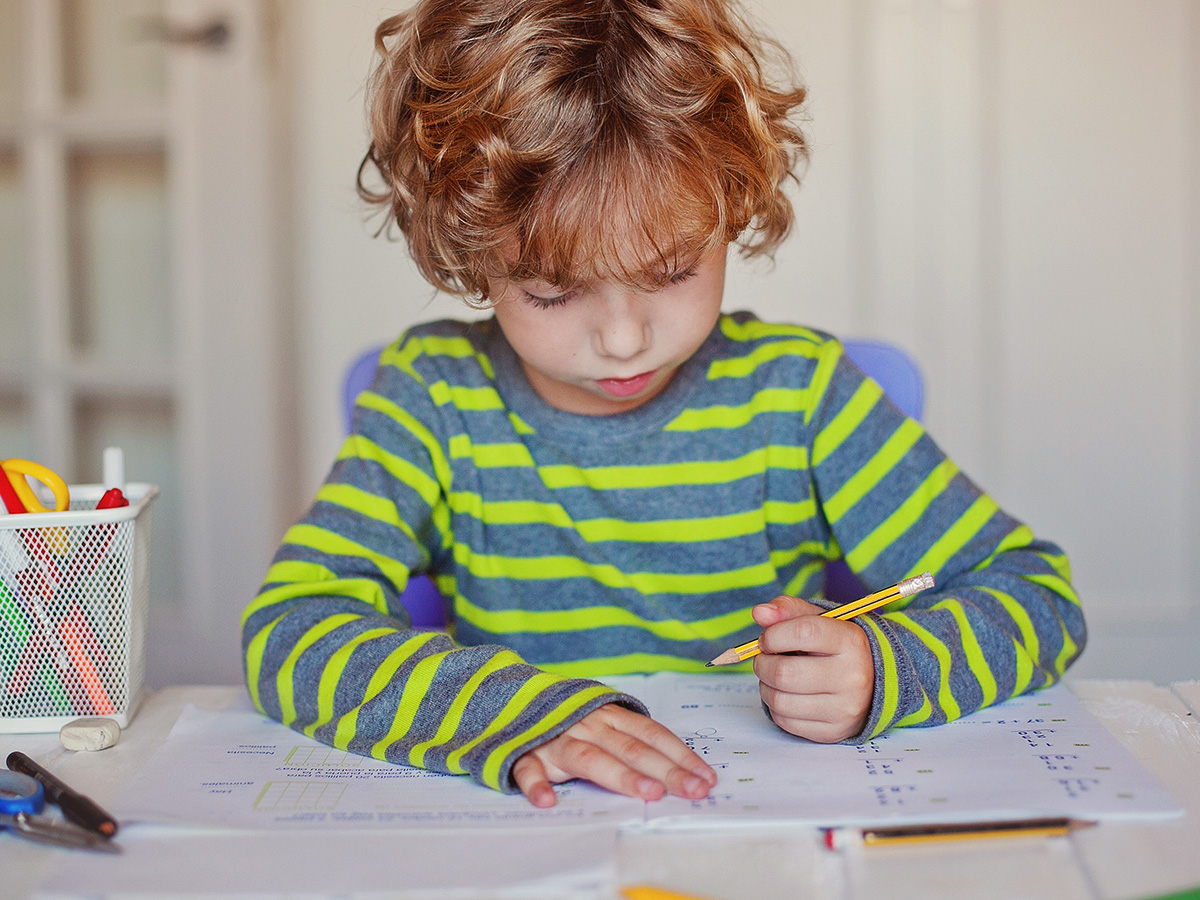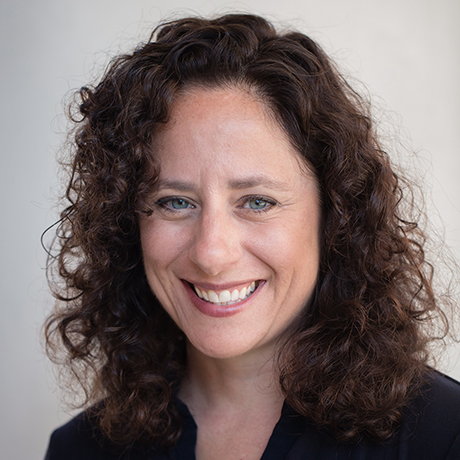# When do kids learn fractions?## ByTara Drinks## At a glance

• Kids start to learn about fractions in first and second grade.

• By the end of grade school, many kids understand and can solve basic problems with fractions. Others need more time.

• Fractions are a difficult math concept, and lots of kids struggle with them.

At some point, every math student has to learn fractions. And for many kids, this can be a real challenge. Math concepts like fractions are difficult — more so than basic math skills like adding and subtracting.

Young kids first run into fractions in everyday life. For example, you might offer half a cookie, or share a drink by pouring it into two equal cups.

Once kids start school, they learn about fractions in stages. Here’s when kids are typically taught:

• Grades 1 and 2: The basic concept of fractions is introduced, with examples like cutting a cake into equal parts.
• Grade 3: The teaching of fractions becomes more formal. Kids learn about numerators and denominators.
• Grade 4: Kids start to work with and compare fractions.
• Grade 5: Kids solve problems with fractions. For example, kids are asked to add and subtract fractions with different denominators, like ¾ + ⅝.

By the end of grade school, many kids understand and can do basic problems with fractions. Others may need more time and practice to fully understand the concept. It’s common for students to have a hard time with fractions — even kids who do well in math.

In middle and high school, kids continue to learn about fractions. But the work gets even more complex. Students may be asked to multiply fractions, like ¾ × ½, or use variables.

When kids are having trouble with fractions, educators and families should work together to understand what’s happening. Teachers may be able to give kids extra attention or practice with fractions — or teach fractions in a different way.

## Share